## Articles in the permanent category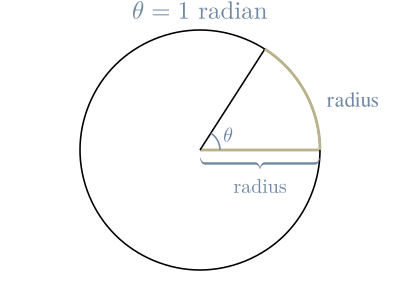A measurement of an angle in radiuses

• ## Matrix Inverse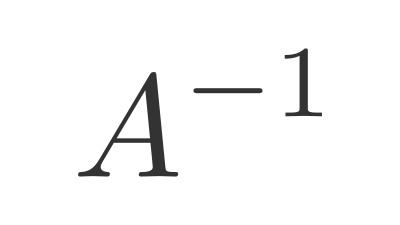A matrix that reverses a transformation.

• ## Identity Matrix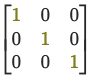When you multiply a matrix $(A)$ by the Identity Matrix $(I)$, you get the original matrix back.

$A …$

• ## Law Of Cosines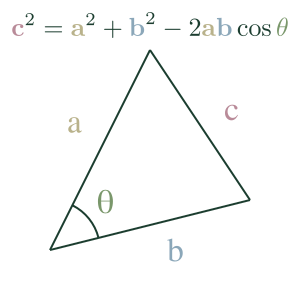The Law of Cosines expresses the relationship between the length of a triangle's sides and one of its angles.

• ## Roblox Vector3

In Roblox, a Vector3 defines a Vector with three components. We use it for setting the position, rotation, and size of elements.

For example, a …

• ## Matrix Multiplication

Matrix multiplication is a mathematical operation between 2 matrices that returns a matrix.

For each row in the first matrix, take the Dot Productf …

• ## Dot Product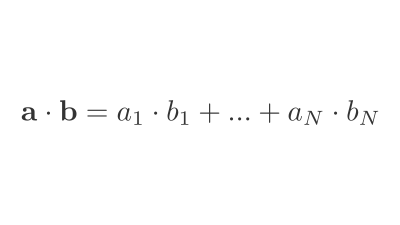An operation between 2 vectors that returns a number.

• ## Binary Cross-Entropy Loss

Binary Cross-Entropy (BCE), also known as log loss, is a loss function used in binary or multi-label machine learning training.

It's nearly identical to Negative …

• ## Presizing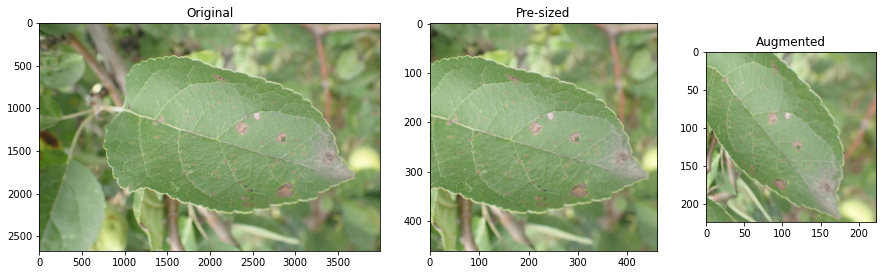Pre-sizing is a technique for preparing images for training a neural network.

In standard image pipelines, images are resized to a size suitable for our …

• ## Categorical Cross-Entropy Loss

Categorical Cross-Entropy Loss Function, also known as Softmax Loss, is a loss function used in multiclass classification model training. It applies the Softmax Activation Function …